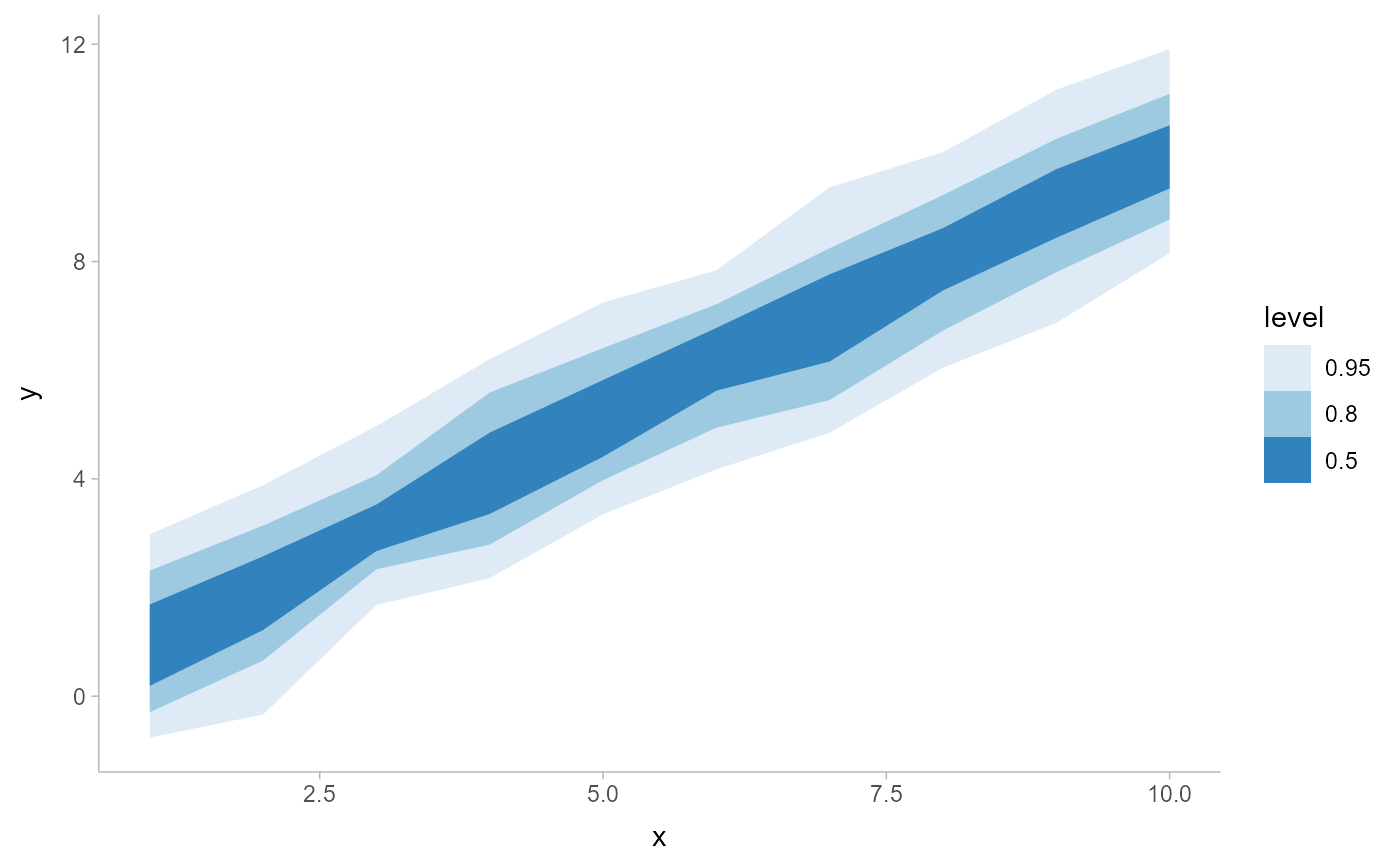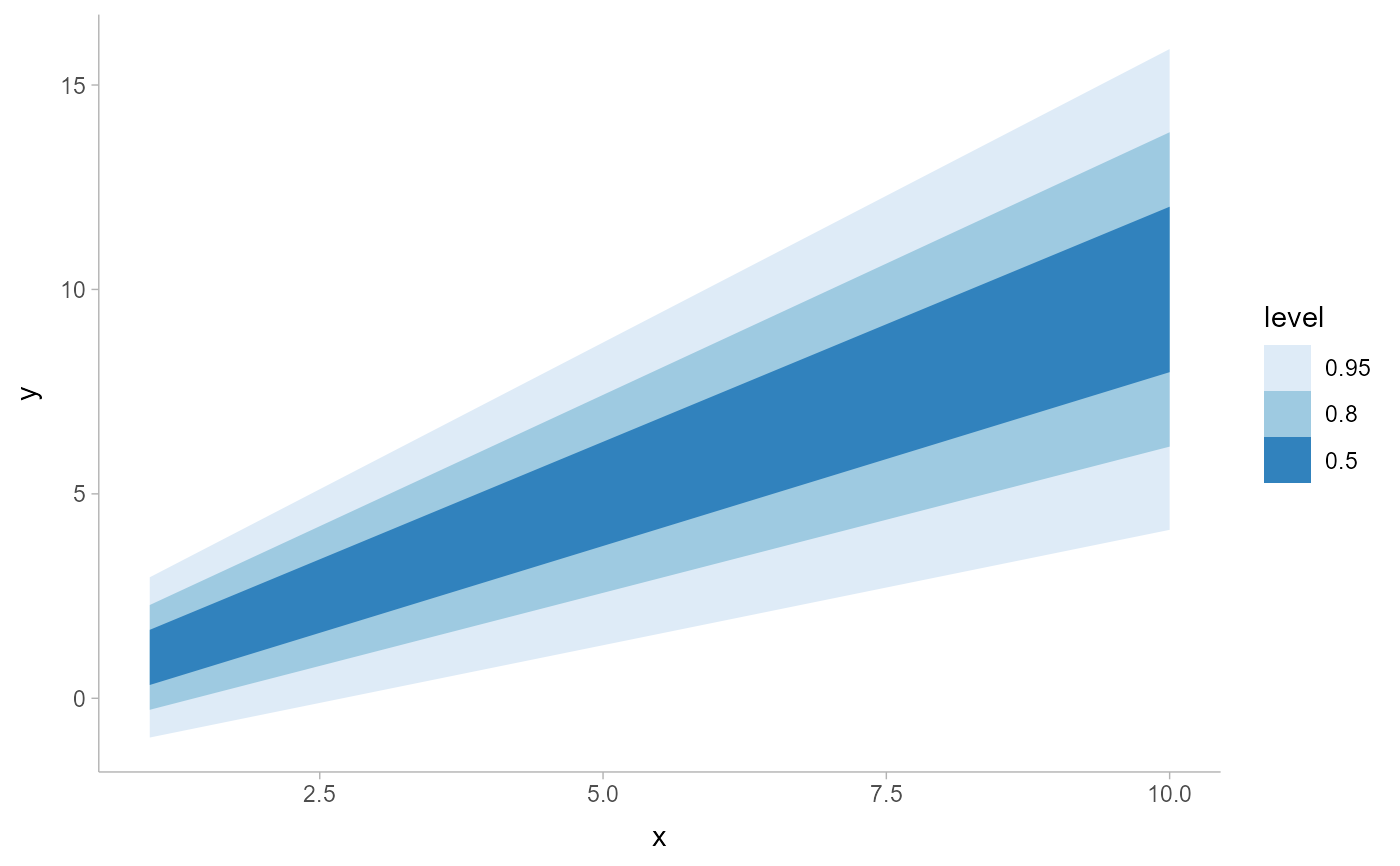A combination of stat_slabinterval() and geom_lineribbon() with sensible defaults for making multiple-ribbon plots. While geom_lineribbon() is intended for use on data frames that have already been summarized using a point_interval() function, stat_ribbon() is intended for use directly on data frames of draws or of analytical distributions, and will perform the summarization using a point_interval() function.

Roughly equivalent to:

stat_lineribbon(

show_point = FALSE

)

stat_ribbon(
mapping = NULL,
data = NULL,
geom = "lineribbon",
position = "identity",
...,
.width = c(0.5, 0.8, 0.95),
point_interval = "median_qi",
orientation = NA,
na.rm = FALSE,
show.legend = NA,
inherit.aes = TRUE
)

## Arguments

mapping

Set of aesthetic mappings created by aes(). If specified and inherit.aes = TRUE (the default), it is combined with the default mapping at the top level of the plot. You must supply mapping if there is no plot mapping.

data

The data to be displayed in this layer. There are three options:

If NULL, the default, the data is inherited from the plot data as specified in the call to ggplot().

A data.frame, or other object, will override the plot data. All objects will be fortified to produce a data frame. See fortify() for which variables will be created.

A function will be called with a single argument, the plot data. The return value must be a data.frame, and will be used as the layer data. A function can be created from a formula (e.g. ~ head(.x, 10)).

geom

Use to override the default connection between stat_ribbon() and geom_lineribbon()

position

Position adjustment, either as a string, or the result of a call to a position adjustment function. Setting this equal to "dodge" (position_dodge()) or "dodgejust" (position_dodgejust()) can be useful if you have overlapping geometries.

...

Other arguments passed to layer(). These are often aesthetics, used to set an aesthetic to a fixed value, like colour = "red" or linewidth = 3 (see Aesthetics, below). They may also be parameters to the paired geom/stat. When paired with the default geom, geom_lineribbon(), these include:

step

Should the line/ribbon be drawn as a step function? One of:

• FALSE (default): do not draw as a step function.

• "mid" (or TRUE): draw steps midway between adjacent x values.

• "hv": draw horizontal-then-vertical steps.

• "vh": draw as vertical-then-horizontal steps.

TRUE is an alias for "mid" because for a step function with ribbons, "mid" is probably what you want (for the other two step approaches the ribbons at either the very first or very last x value will not be visible).

.width

The .width argument passed to point_interval: a vector of probabilities to use that determine the widths of the resulting intervals. If multiple probabilities are provided, multiple intervals per group are generated, each with a different probability interval (and value of the corresponding .width and level generated variables).

point_interval

A function from the point_interval() family (e.g., median_qi, mean_qi, mode_hdi, etc), or a string giving the name of a function from that family (e.g., "median_qi", "mean_qi", "mode_hdi", etc; if a string, the caller's environment is searched for the function, followed by the ggdist environment). This function determines the point summary (typically mean, median, or mode) and interval type (quantile interval, qi; highest-density interval, hdi; or highest-density continuous interval, hdci). Output will be converted to the appropriate x- or y-based aesthetics depending on the value of orientation. See the point_interval() family of functions for more information.

orientation

Whether this geom is drawn horizontally or vertically. One of:

• NA (default): automatically detect the orientation based on how the aesthetics are assigned. Automatic detection works most of the time.

• "horizontal" (or "y"): draw horizontally, using the y aesthetic to identify different groups. For each group, uses the x, xmin, xmax, and thickness aesthetics to draw points, intervals, and slabs.

• "vertical" (or "x"): draw vertically, using the x aesthetic to identify different groups. For each group, uses the y, ymin, ymax, and thickness aesthetics to draw points, intervals, and slabs.

For compatibility with the base ggplot naming scheme for orientation, "x" can be used as an alias for "vertical" and "y" as an alias for "horizontal" (ggdist had an orientation parameter before base ggplot did, hence the discrepancy).

na.rm

If FALSE, the default, missing values are removed with a warning. If TRUE, missing values are silently removed.

show.legend

Should this layer be included in the legends? NA, the default, includes if any aesthetics are mapped. FALSE never includes, and TRUE always includes.

inherit.aes

If FALSE, overrides the default aesthetics, rather than combining with them. This is most useful for helper functions that define both data and aesthetics and shouldn't inherit behaviour from the default plot specification, e.g. borders().

## Value

A ggplot2::Stat representing a multiple-ribbon geometry which can be added to a ggplot() object.

## Details

To visualize sample data, such as a data distribution, samples from a bootstrap distribution, or a Bayesian posterior, you can supply samples to the x or y aesthetic.

To visualize analytical distributions, you can use the xdist or ydist aesthetic. For historical reasons, you can also use dist to specify the distribution, though this is not recommended as it does not work as well with orientation detection. These aesthetics can be used as follows:

• xdist, ydist, and dist can be any distribution object from the distributional package (dist_normal(), dist_beta(), etc) or can be a posterior::rvar() object. Since these functions are vectorized, other columns can be passed directly to them in an aes() specification; e.g. aes(dist = dist_normal(mu, sigma)) will work if mu and sigma are columns in the input data frame.

• dist can be a character vector giving the distribution name. Then the arg1, ... arg9 aesthetics (or args as a list column) specify distribution arguments. Distribution names should correspond to R functions that have "p", "q", and "d" functions; e.g. "norm" is a valid distribution name because R defines the pnorm(), qnorm(), and dnorm() functions for Normal distributions.

See the parse_dist() function for a useful way to generate dist and args values from human-readable distribution specs (like "normal(0,1)"). Such specs are also produced by other packages (like the brms::get_prior function in brms); thus, parse_dist() combined with the stats described here can help you visualize the output of those functions.

## Computed Variables

The following variables are computed by this stat and made available for use in aesthetic specifications (aes()) using the after_stat() function or the after_stat argument of stage():

• x or y: For slabs, the input values to the slab function. For intervals, the point summary from the interval function. Whether it is x or y depends on orientation

• xmin or ymin: For intervals, the lower end of the interval from the interval function.

• xmax or ymax: For intervals, the upper end of the interval from the interval function.

• .width: For intervals, the interval width as a numeric value in [0, 1]. For slabs, the width of the smallest interval containing that value of the slab.

• level: For intervals, the interval width as an ordered factor. For slabs, the level of the smallest interval containing that value of the slab.

• pdf: For slabs, the probability density function (PDF). If options("ggdist.experimental.slab_data_in_intervals") is TRUE: For intervals, the PDF at the point summary; intervals also have pdf_min and pdf_max for the PDF at the lower and upper ends of the interval.

• cdf: For slabs, the cumulative distribution function. If options("ggdist.experimental.slab_data_in_intervals") is TRUE: For intervals, the CDF at the point summary; intervals also have cdf_min and cdf_max for the CDF at the lower and upper ends of the interval.

## Aesthetics

The line+ribbon stats and geoms have a wide variety of aesthetics that control the appearance of their two sub-geometries: the line and the ribbon.

These stats support the following aesthetics:

• x: x position of the geometry (when orientation = "vertical"); or sample data to be summarized (when orientation = "horizontal" with sample data).

• y: y position of the geometry (when orientation = "horizontal"); or sample data to be summarized (when orientation = "vertical" with sample data).

• xdist: When using analytical distributions, distribution to map on the x axis: a distributional object (e.g. dist_normal()) or a posterior::rvar() object.

• ydist: When using analytical distributions, distribution to map on the y axis: a distributional object (e.g. dist_normal()) or a posterior::rvar() object.

• dist: When using analytical distributions, a name of a distribution (e.g. "norm"), a distributional object (e.g. dist_normal()), or a posterior::rvar() object. See Details.

• args: Distribution arguments (args or arg1, ... arg9). See Details.

In addition, in their default configuration (paired with geom_lineribbon()) the following aesthetics are supported by the underlying geom:

Ribbon-specific aesthetics

• xmin: Left edge of the ribbon sub-geometry (if orientation = "horizontal").

• xmax: Right edge of the ribbon sub-geometry (if orientation = "horizontal").

• ymin: Lower edge of the ribbon sub-geometry (if orientation = "vertical").

• ymax: Upper edge of the ribbon sub-geometry (if orientation = "vertical").

• order: The order in which ribbons are drawn. Ribbons with the smallest mean value of order are drawn first (i.e., will be drawn below ribbons with larger mean values of order). If order is not supplied to geom_lineribbon(), -abs(xmax - xmin) or -abs(ymax - ymax) (depending on orientation) is used, having the effect of drawing the widest (on average) ribbons on the bottom. stat_lineribbon() uses order = after_stat(level) by default, causing the ribbons generated from the largest .width to be drawn on the bottom.

Color aesthetics

• colour: (or color) The color of the line sub-geometry.

• fill: The fill color of the ribbon sub-geometry.

• alpha: The opacity of the line and ribbon sub-geometries.

• fill_ramp: A secondary scale that modifies the fill scale to "ramp" to another color. See scale_fill_ramp() for examples.

Other aesthetics (these work as in standard geoms)

• group

See examples of some of these aesthetics in action in vignette("lineribbon"). Learn more about the sub-geom override aesthetics (like interval_color) in the scales documentation. Learn more about basic ggplot aesthetics in vignette("ggplot2-specs").

See geom_lineribbon() for the geom underlying this stat.

Other lineribbon stats: stat_lineribbon()

## Examples

library(dplyr)
library(ggplot2)
library(distributional)

theme_set(theme_ggdist())

# ON SAMPLE DATA
tibble(x = 1:10) %>%
group_by_all() %>%
do(tibble(y = rnorm(100, .\$x))) %>%
ggplot(aes(x = x, y = y)) +
stat_ribbon() +
scale_fill_brewer()# ON ANALYTICAL DISTRIBUTIONS
# Vectorized distribution types, like distributional::dist_normal()
# and posterior::rvar(), can be used with the xdist / ydist aesthetics
tibble(
x = 1:10,
sd = seq(1, 3, length.out = 10)
) %>%
ggplot(aes(x = x, ydist = dist_normal(x, sd))) +
stat_ribbon() +
scale_fill_brewer()